# [RitSec CTF] soup

Can we guess the favoutite Soup of the creator of these challenge? Let’s discover it!

What we’re provided with is an ELF 64-bit file which once executed, without arguments will tell us which is the correct usage:

`"Usage: ./soup <soup> <L33t_S0UP>"`

but nothing is told about what these two arguments realli are: let’s analyze them with IDA then! Skipping the boring things (checks on the number of arguments for example), we can focus on this function: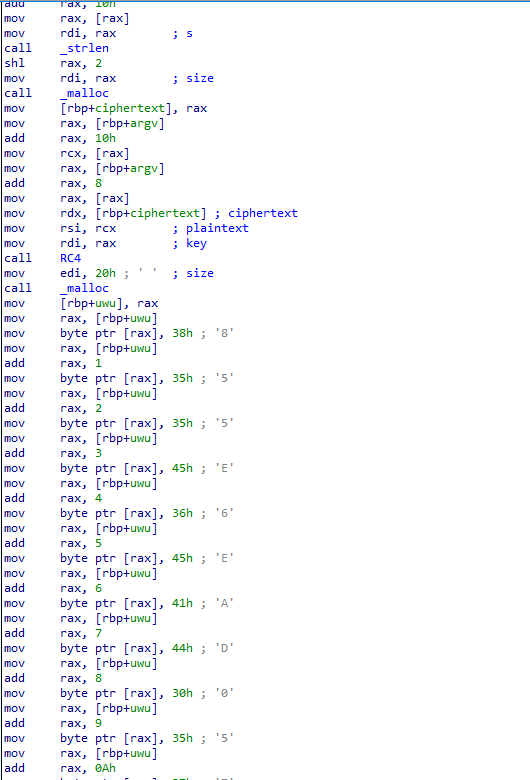And see what do we have here: we have a function I haven’t seen until now: RC4. It takes 3 arguments: ciphertext, plaintext and key (could they be the arguments we’ve to insert while executing this program?). And right after it, I can see it is gradually forming a string. What is it useful for? Let’s generate the pseudocode to have it clearer: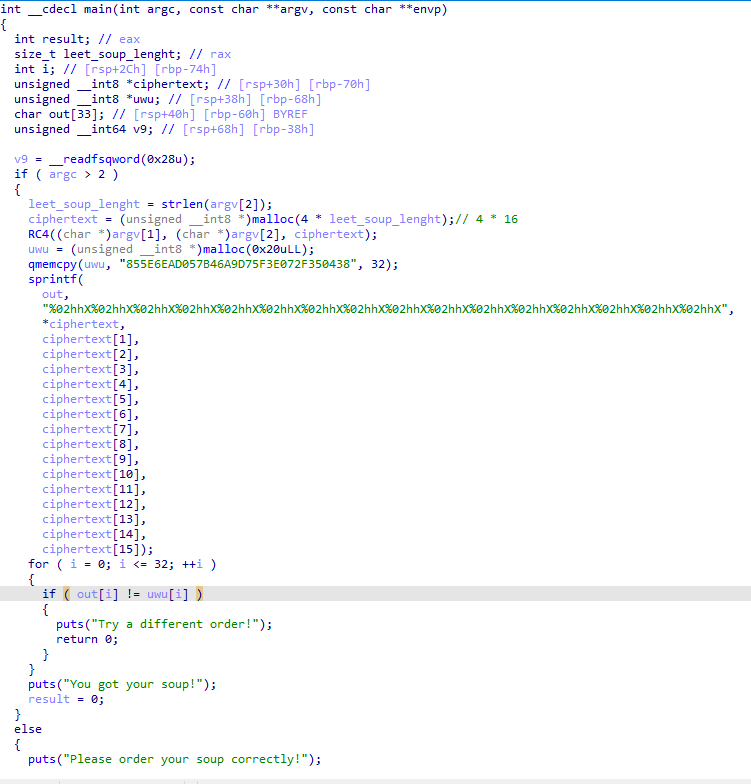Now that that’s clearer let’s make a picture of its functionalities:

• we have an RC4 function that takes as arguments argv (key), argv (plaintext), and ciphertext that at the moment is empty;
• it copies the hexadecimal string “855E6EAD057B46A9D75F3E072F350438” into the variable uwu;
• it generates the variable out, converting the result contained in ciphertext variable in an hexadecimal string;
• It compares uwu and out: if they’re equal, “we have our soup”.

So we’ve understood that we have to insert the right key and the input that generate the supposed output. Let’s reverse the function that does that: RC4.

RC4 is the Rivest Cipher 4 and it is composed by two phases:

• Key-scheduling algorithm (KSA): it uses the key to generate an S-box that is later used from the PRGA function;
•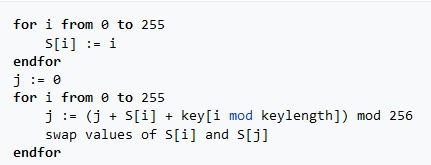• Pseudo-random generation algorithm (PRGA): it uses the previously generated S-box and the plaintext to generate an output K that finally is XORed with the plaintext to actually generate the ciphertext.
•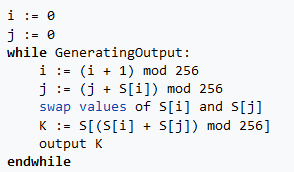Well now: we don’t even need to reverse it, since it is a XOR we know that given C as ciphertext, K as key and P as plaintext:

• M xor K = C
• C xor K = M

We have the ciphertext we want to obtain, we have to generate the plaintext we would need to obtain that, and succeed in the check the program does, but we’re missing the key! So let’s just write the program to generate the desired input:

``````output_str=[]
outhex=""
hexastring=""
shex=[]
outascii=""
S=[]

key="soup"
input_str=[133, 94, 110, 173, 5, 123, 70, 169, 215, 95, 62, 7, 47, 53, 4, 56]
#it is just the hex for the above array : input_str=["85" , "5E", "6E", "AD", "05","7B","46","A9","D7","5F","3E","07","2F","35", "04", "38"]

input_len=len(input_str)
keylength=len(key)

#KSA
for i in range(256):
S.append(i)
j = 0
for i  in range (256):
j = (j + S[i] + ord(key[i%keylength]))% 256
S[i], S[j] = S[j], S[i]

#PRGA
i = 0
j = 0
for l in range (input_len):
i = (i + 1) % 256
j = (j + S[i]) % 256
S[i], S[j] = S[j], S[i]
output_str.append(S[(S[i] + S[j])%256] ^ input_str[l])

for hexa in S:
shex.append(hex(hexa))

#Print Section
print ("S: ", shex)
print ("Input: ",input_str)
print ("Key: ", key)
print ("Output: ", output_str)
for out in output_str:
outhex+=str(hex(out))
outascii+=chr(out)
print ("Hex Output: ", outhex.replace("0x", ""))
print ("Decoded Output: ", outascii)
``````

Surely not the most elegant script but it does what it is supposed to do. It generates the needed input to obtain the scripted output, given a KEY: that’s the last part given.

It is a guessy part of the challenge, or at least I haven’t found anything that could help me to get the right key or at least its lenght into the disassembled code, so after trying a random key like “supersecurekey”, I’ve decided to try the most trivial one I could that had a relation with the challenge: soup.

In the end it was the output of my script: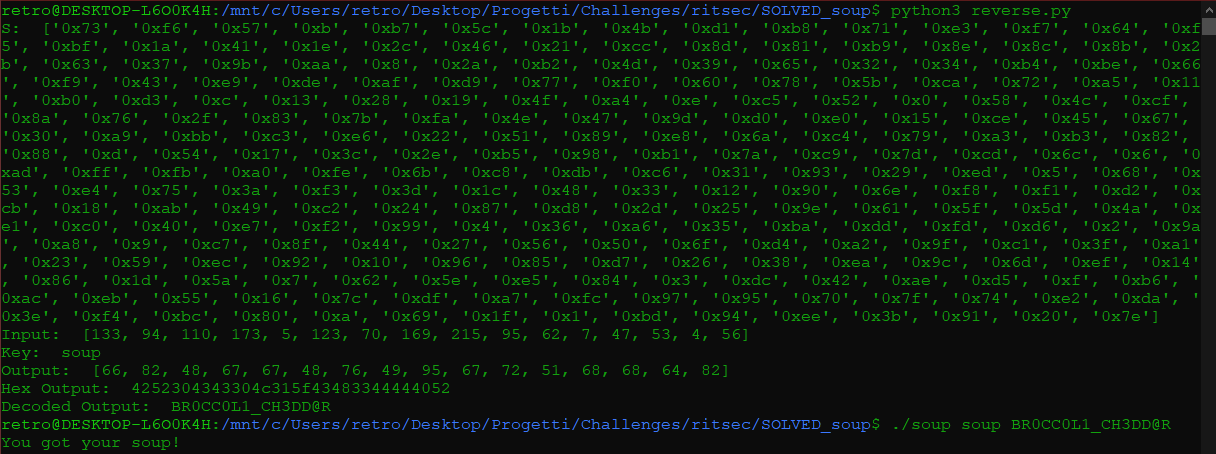Well, here we are. At this point we only had to put the flag in its correct format and submit it:

`RS{BR0CC0L1_CH3DD@R}`